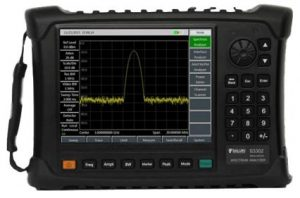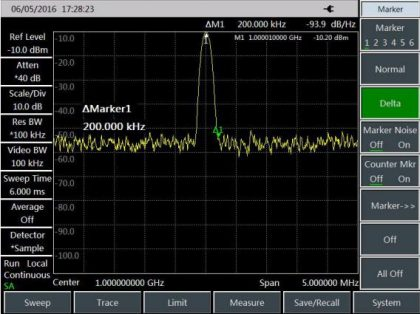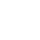CurrentHome>Resources
Noise Signal Measurement of S3302 Handheld Spectrum Analyzer (Part I)
Release time :2021.03.23 Reading quantity:47

Saluki S3302 series handheld spectrum analyzer has a variety of measurement functions. One of the measurement methods is noise signal measurement. This section we will introduce this measurement by two parts.Fig.1 Saluki S3302 series handheld spectrum analyzer

1. Definition of noise signal

In communications systems, signal-to-noise ratio (SNR) is usually used to express noise amplitude. When the nose level in the system increases, SNR decreases and it will be harder to demodulate modulated signals. SNR measurement is also used to indicate measurement of ratio of carrier against noise in communications systems.

Measurement of the S/N and noise by the marker function of S3302 series spectrum analyzer is described below. In the example, SNR is measured with the signal (carrier) having only single frequency point. If the modulation signal is tested, this test process should be modified to correct the level of the modulation signal.

2. Measure SNR

a) Set the output signal of the signal generator:

Set the frequency of the signal generator as 1GHz and power as -10dBm. Connect the output of the signal generator to the input port of the spectrum analyzer, as shown in Fig. 2. Enable the ON state of the radio frequency.

b) Set the center frequency, span, reference level and attenuator.

Press [Reset].

Press [Frequency], [Center Frequency], 1[GHz].

Press [Frequency], [Span] and 5[MHz].

Press [Amplitude], [Ref Level] and -10[dBm].

Press [Amplitude], [Atten Auto Man] and 40[dB].

c) Set the marker at the signal peak, and the differential marker at the noise location with the offset of 200kHz.

Press [Peak] and [Peak Track Off On].

Press [Marker], [Delta] and 200[kHz].

d) Enable the noise marker function and observe the S/N:

Press [Marker] and [Noise Marker Off On].

As shown in Fig. 2, the S/N reading is in dBc/Hz, as the noise value refers to the noise bandwidth normalized to 1Hz.

This value decreases by 10×log(BW). If you wish to obtain noise values under different channel bandwidth, the measurement result needs to be corrected based on current bandwidth. For example, if the reading of the spectrum analyzer is -85dBc/Hz and the channel bandwidth is bandwidth, S/N is:

S/N=85dBc/Hz – 10×log(30kHz) =40.2dBc/(30kHz)

If the differential marker is less than one fourth of the edge distance between the signal peak and response, errors may occur in noise measurement.Fig. 2  S/N Measurement